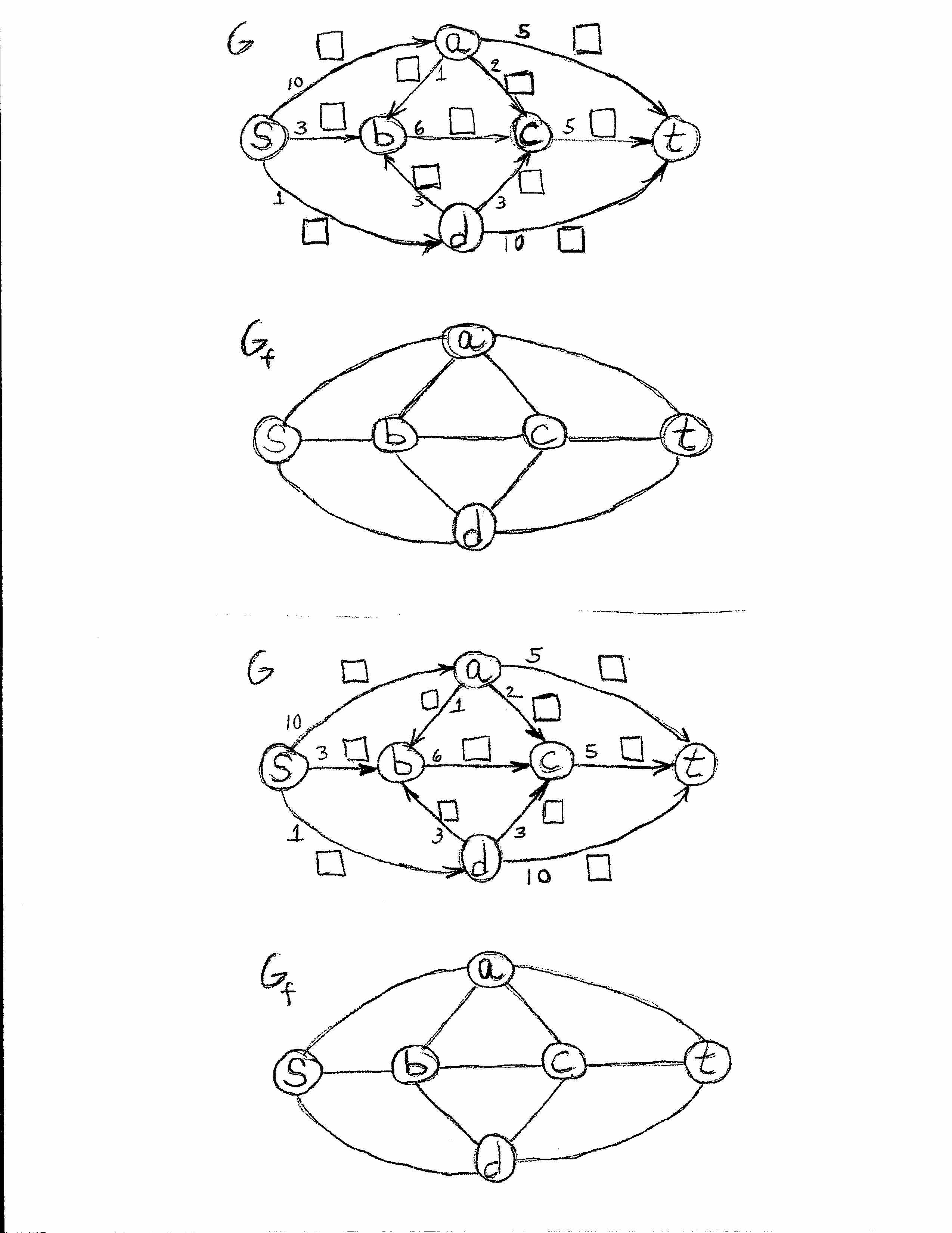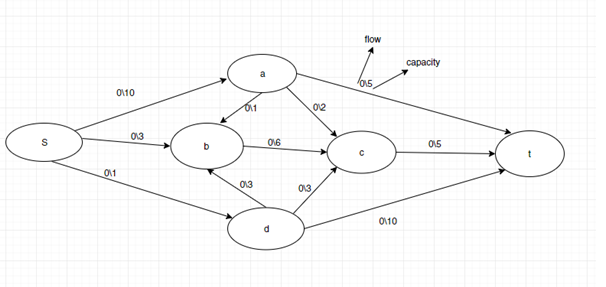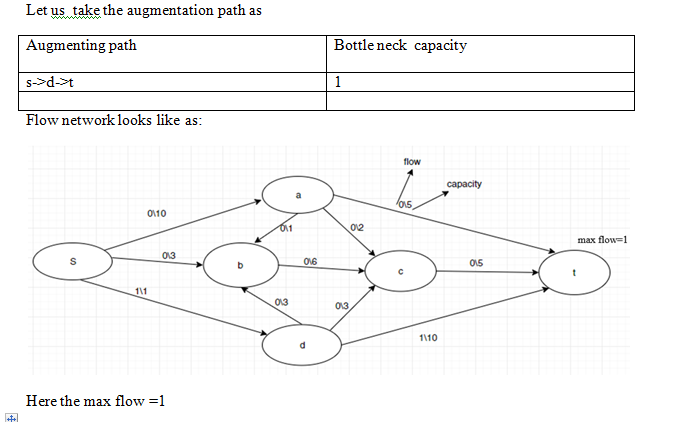2(033G,G D98ヘく囗1051 0

Question

Use the worksheets to show, one path augmentation at a time, how to use the Ford-Fulkerson Algorithm to compute a max flow and a min cut for the flow network.

As you go along, write the flows for each edge in the little squares. When you reach the end of the algorithm, shade in the nodes that are on the "A side" of the minimum cut.

You may want to review the Ford-Fulkerson Algorithm before starting on the problem.help_outlineImage Transcriptionclose2 (0 3 3 G, G D 98ヘく囗 10 5 1 0 fullscreen
Step 1

For solving your problem first of all we define Ford-Fulkerson Algorithm.

Ford-Fulkerson Algorithm for Maximum Flow Problem:-

Given a graph which represents a flow network where every edge has a capacity. Also given two vertices source ‘s’ and sink ‘t’ in the graph, find the maximum possible flow from s to t with following constraints:

1. a)Flow on an edge doesn’t exceed the given capacity of the edge.
2. b)Incoming flow is equal to outgoing flow for every vertex except s and t.

A path with available capacity is called an augmenting path.

The max-flow min-cut  states that in a flow network, the maximum amount of flow passing from the source to the sink is equal to the total weight of the edges in the minimum cut, i.e. the smallest total weight of the edges which if removed would disconnect the source from the sink.

Step 2

The capacity of each edge is given in the graph, s is a source and t is a terminated point

Following shows the first steps of Ford–Fulkerson in a flow network with 6 nodes, source s and sink t.

Let us solve this problem step by step:

Initially max flow=0Step 3...

Want to see the full answer?

See Solution

Want to see this answer and more?

Our solutions are written by experts, many with advanced degrees, and available 24/7

See Solution
Tagged in

Computer Science